# Constructing a Circle Through Three Given Points

Compass and straight edge constructions are of interest to mathematicians, not only in the field of geometry, but also in algebra. For thousands of years, beginning with the Ancient Babylonians, mathematicians were interested in the problem of "squaring the circle" (drawing a square with the same area as a circle) using a straight edge and compass. This problem is equivalent to finding the area of a circle. It turns out that this is impossible, but no-one managed to prove this until 1882!

However, it is possible to construct a circle through three given points using only a straight edge and compass. For this construction, you will need a straight edge (ruler - but you won't be measuring anything), a pair of compasses, a pencil and paper. I have drawn the pictures using the robocompass app. It's fun to play with, and you can use it to do all sorts of geometric constructions. There's a little bit of coding to learn, but a list of instructions is provided. Once you've written your little program, you can invite a few friends over to watch the construction. Be warned that, when you are using it to draw arcs, the robocompass compass point may appear to be a little away from the centre, but the drawing is actually accurate.

Note: these instructions assume that you are familiar with constructing the perpendicular bisector of a straight line. We are going to do that twice. If your memories of this construction are a bit hazy, it's probably a good idea to re-read the article on finding the perpendicular bisector of a line segment before trying to do this construction.

## The Construction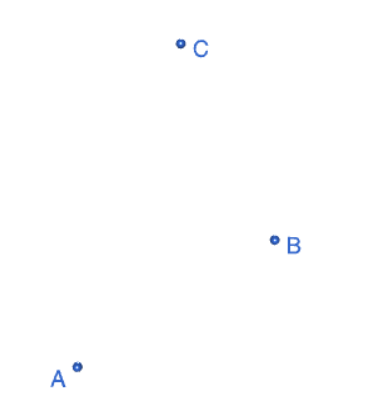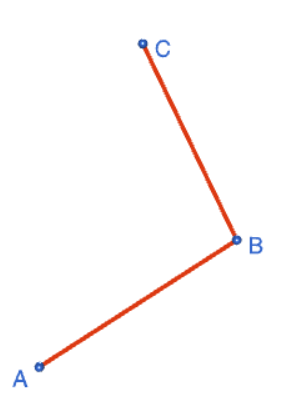Step 2: This step is optional. If you think it will help you, join up line segments $AB$ and $BC$. These are the line segments that we are going to bisect to help us find the centre of our circle.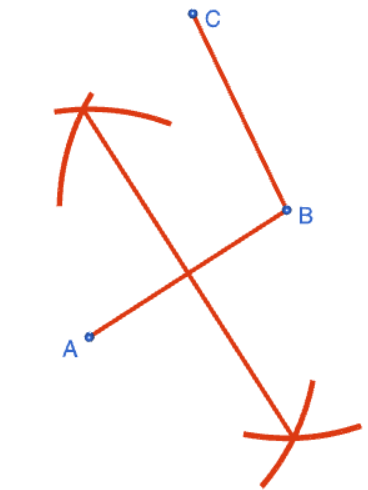Step 3: Construct the perpendicular bisector of line segment $AB$. Make it nice and long so that it will intersect the line segment drawn in the next step.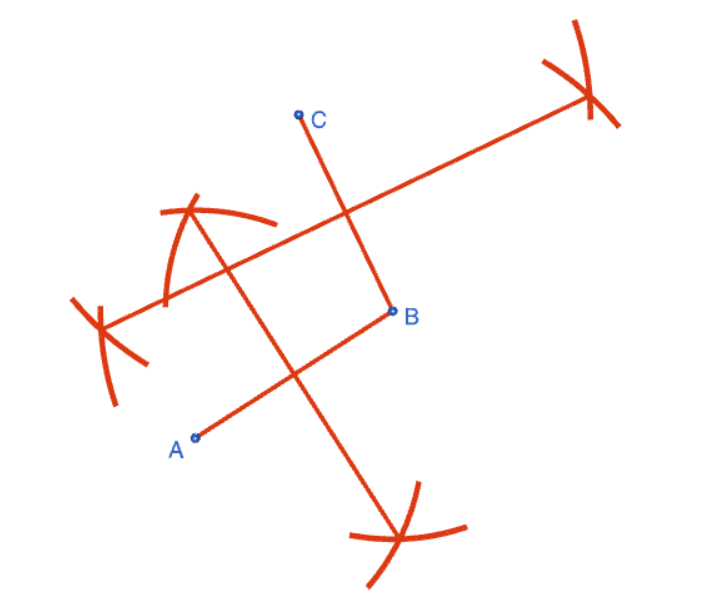Step 4: Construct the perpendicular bisector of line segment $BC$. Make it long enough the intersect the perpendicular bisector drawn in step 3. Label the point where the two perpendicular bisectors intersect with an $O$. This will be the centre of our circle.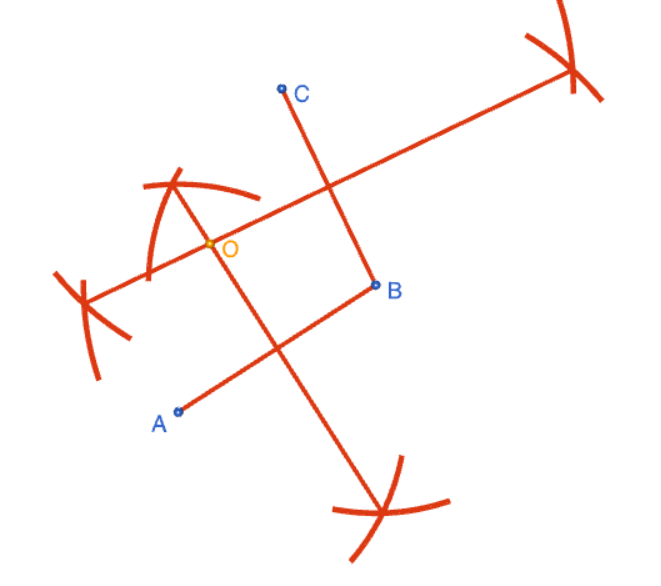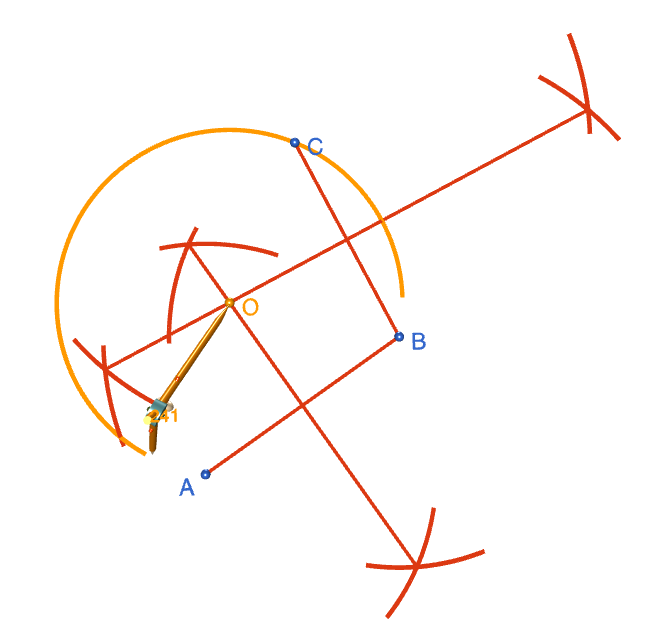Step 5: Place the tip of the compasses at the point $O$. Open them out so that the pencil touches one of points $A$, $B$ or $C$. It doesn't matter which: they're all the same distance away from point $O$. Draw a circle of this radius. Notice how it passes through all three points? The completed construction is shown on the right. Sit back and admire your art work. Tell any one who will listen that it should be in the Louvre!

What's that, Sam? Your circle is squashed? That's probably because you didn't use a ruler to draw your line segments.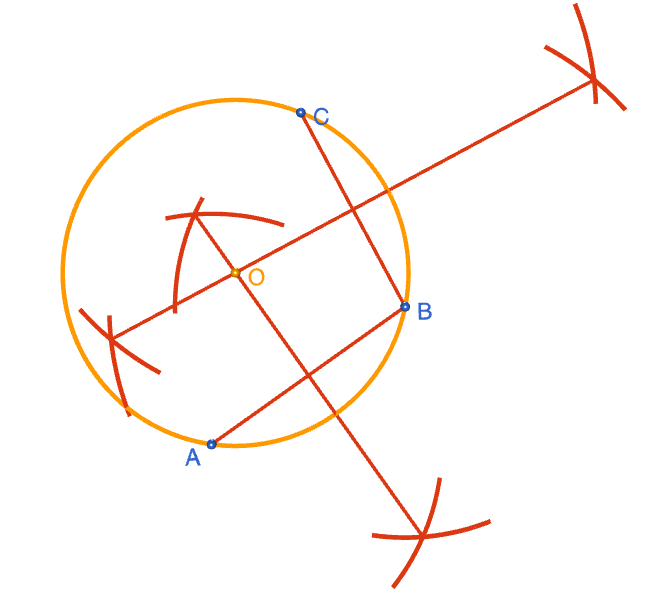### Description

This tutorial will expose you to Rulers and how to use them. We will also have a close look as set square and compass constructions. Your feedback is important to us, if you like any other topic covered under this tutorial, please do let us know.

### Audience

Year 10 or higher

### Learning Objectives

Ruler and compass constructions and more

Author: Subject Coach
You must be logged in as Student to ask a Question.